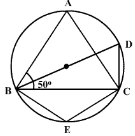## MCQ Questions for Class 9 Maths: Ch 10 Circles1. The region between an arc and the two radii joining the centre of the end points of the arc is called a:
(a) Segment
(b) Semi circle
(c) Minor arc
(d) Sector
► (d) Sector

2. In a circle with center O and a chord BC, the point D lies on the same side BC as O. If ∠ BOC = 50°,then ∠ BDC =
(a) 25°
(b) 100°
(c) 75°
(d) 150°
► (a) 25°

3. The region between chord and either of the arc is called
(a) a sector
(b) a semicircle
(c) a segment
(d) a quarter circle
► (c) a segment

4. AB is a chord of a circle with radius ‘r’. If P is any point on the circle such that ∠APB is a right angle , then AB is equal to
(a) 3r
(b) r
(c) 2r
(d) r2
► (c) 2r

5. In the figure, triangle ABC is an isosceles triangle with AB = AC and measure of angle ABC = 50°. Then the measure of angle BDC and angle BEC will be(a) 60°, 100°
(b) 80°, 100°
(c) 50°, 100°
(d) 40°, 120°
► (b) 80°, 100°

6. In the given figure if OA = 5 cm, AB = 8 cm and OD is perpendicular to AB, then CD is equal to(a) 4 cm
(b) 3 cm
(c) 5 cm
(d) 2 cm
► (d) 2 cm

7. Segment of a circle is the region between an arc and ………..of the circle.
(a) perpendicular
(c) chord
(d) secant
► (c) chord

8. The degree measure of a semicircle is
(a) 0°
(b) 90°
(c) 360°
(d) 180°
► (d) 180°

9. If chords AB and CD of congruent circles subtend equal angles at their centres, then:
(a) AB = CD
(b) AB > CD
(d) None of the above
► (a) AB = CD

10. The angle subtended by the diameter of a semi-circle is:
(a) 90
(b) 45
(c) 180
(d) 60
► (c) 180

11. The center of the circle lies in______ of the circle.
(a) Interior
(b) Exterior
(c) Circumference
(d) None of the above
► (a) Interior

12. Equal _____ of the congruent circles subtend equal angles at the centers.
(a) Segments
(c) Arcs
(d) Chords
► (d) Chords

13. In a circle with center O and a chord BC, points D and E lie on the same side of BC. Then, if∠BDC=80°, then ∠BEC =
(a) 80°
(b) 20°
(c) 160°
(d) 40°
►
A.
80°

14. A regular octagon is inscribed in a circle. The angle that each side of the octagon subtends at the centre is
(a) 45°
(b) 75°
(c) 90°
(d) 60°
►
A.
45°

15. AD is diameter of a circle and AB is a chord. If AD = 50 cm, AB = 48 cm, then the distance of AB from the centre of the circle is
(a) 6 cm
(b) 8 cm
(c) 5 cm
(d) 7 cm
► (d) 7 cm

16. A chord of a circle which is twice as long as its radius is a ____ of the circle
(a) Diameter
(b) perpendicular
(c) arc
(d) secant
► (a) Diameter

17. If there are two separate circles drawn apart from each other, then the maximum number of common points they have:
(a) 0
(b) 1
(c) 2
(d) 3
► (a) 0

18. If a line intersects two concentric circles with centre O at A, B, C and D, then:
(a) AB = CD
(b) AB > CD
(c) AB < CD
(d) None of the above
► (a) AB = CD